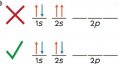# half integer spin meaning of fermions

Joined May 11, 2018
158
Hello what does half integer spin of fermions and full integer spin of bosons means .

i know that from pauli principle , that a matter can not have quantum numbers at the same time . meaning in a single state you can not have spin up and spin down like in the image below .does it mean that fermions only have a spin up only?( and is that the meaning of the electron )
- like, can you say that the 1s has 2 electrons ?

and does it also mean the bosons have both spin up and spin down ? ( is that the meaning of a photon)

#### Papabravo

Joined Feb 24, 2006
20,369
There are generally multiple quantum numbers assigned to particles. The principles says that two particles cannot have all of their quantum numbers the same. So for example 2s electrons, of which there are two in that shell, have the same principle quantum number which is 2, and the the same angular momentum quantum number, s, and the have opposite spin quantum numbers, +1/2 and -1/2. It is the spin angular momentum quantum numbers being different that allows them to occupy the same energy level.

•Joined May 11, 2018
158
ok now where does the difference between fermions and bosons come from !! according to that exaplanation in terms of spin

in other words what does half integer and full integer spin mean. does it have any similarity with quantum number spin

There are generally multiple quantum numbers assigned to particles. The principles says that two particles cannot have all of their quantum numbers the same. So for example 2s electrons, of which there are two in that shell, have the same principle quantum number which is 2, and the the same angular momentum quantum number, s, and the have opposite spin quantum numbers, +1/2 and -1/2. It is the spin angular momentum quantum numbers being different that allows them to occupy the same energy level.

Last edited:

#### Papabravo

Joined Feb 24, 2006
20,369
ok now where does the difference between fermions and bosons come from !! according to that exaplanation in terms of spin

in other words what does half integer and full integer spin mean. does it have any similarity with quantum number spin
So fermions and bosons are fundamentally different kinds of things. The values of their quantum numbers don't follow the same rules.
If an object has half integer spin it is a fermion and follows fermion rules.
If an object has integer spin it is a boson and follows boson rules, aka statistics. This terminology is used in Fermi-Dirac statistics, and Bose-Einstein statistics. There are no known particles of any other type in the universe.

•Joined May 11, 2018
158
So fermions and bosons are fundamentally different kinds of things. The values of their quantum numbers don't follow the same rules.
If an object has half integer spin it is a fermion and follows fermion rules.
If an object has integer spin it is a boson and follows boson rules, aka statistics. This terminology is used in Fermi-Dirac statistics, and Bose-Einstein statistics. There are no known particles of any other type in the universe.

oooh ok i understand but what does half integer spin and full integer spin mean . but anyways thanks for the answers

#### bogosort

Joined Sep 24, 2011
696
oooh ok i understand but what does half integer spin and full integer spin mean . but anyways thanks for the answers
Experimentally (see the Stern-Gerlach experiment), physicists found that quantum particles have a degree of freedom beyond energy, momentum, position, etc. that they called "spin". Different types of particles have different spin, so it was useful to classify the particles by their spin. It turns out that spin is quantized, i.e., it is always some value n/2 for integer n. For n = 1, we have the fermions, or spin-1/2 particles; when n is zero or even, we have the bosons (integer spin). As Papabravo said, the physical characteristics of fermions are associated with matter: atoms are made of quarks and electrons, which are fermions. The physical characteristics of bosons are associated with interactions ("forces"). The most famous bosons are the photon (spin-1) and the Higgs (spin-0).

#### Papabravo

Joined Feb 24, 2006
20,369
Although quantum particles do not move like planets in orbits, it is useful to think of "spin" as if it were a kind of angular momentum, in the same way as electrons move around the nucleus in "orbitals" each with it's own quantized angular momentum.

•